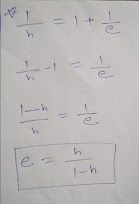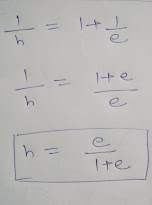### Relationship Between Void Ratio and Porosity

the porosity and void ratio both indicates the void hole in a soil mass but it a different as we discuss in three phase diagram👈 of volumetric relation. the voids ratio define how much voids present as compared to total solids in soil mass, where porosity say how much voids as compared to total volume of soil mass, in void ratio if the void increase in soil mass only top value change. but in porosity both top and bottom value change.👈 there is a relation between void ratio and porosity which very important in soil problem discuss below.

## The relation between porosity and void ratio

we know formula of void ratio (e) = Vv / Vs

in which Vv = Volume of Voids and Vs = Volume of Solids
Porosity formula (n) = Vv / V
Vv = Volume of Voids and V= Total Volume of soil mass.

first letter V represent volume and second letter
a= air, W= water S= solid

also the volume of voids = Vw + Va

Now finding the relation between (e) and (n)

we know value of n, Firstly writes its

Now we reverse the equation by dividing by 1

total volume come upward due to divide

putting total volume V = Vv + Vs

now right side value may be written as shown, we get 2 value on the right side first one is same on lower and above so it gives 1.Also we know Vv / Vs is void ratio and our value is just it reverse

putting e which divide by 1 so our value as according to the equation

star mark equation is most important you can find e from n and n from using  this equation.

firstly finding e value from n

first transfer the 1 from the right side to the left side

then take l cm as shown in fig

the last step is lust reverse the value which give our final equation

we can also find an equation where n is find by ejust write the stat mark equation

an instant of transfer 1 toward left side this time

just take l cm of right side equation

now simply reverse the equation

and we ger a equation where you find porosity by using void ratio only.